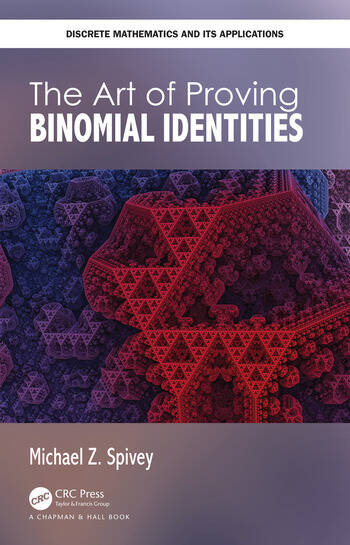The Art of Proving Binomial Identities

1st Edition

Michael Z. Spivey

Chapman and Hall/CRC
Published May 14, 2019
Textbook - 368 Pages - 29 B/W Illustrations
ISBN 9780815379423 - CAT# K339100
Series: Discrete Mathematics and Its Applications

For Instructors Request Inspection Copy

was \$89.95

USD\$71.96

SAVE ~\$17.99

FREE Standard Shipping!

Preview

Summary

The Art of Proving Binomial Identities accomplishes two goals: (1) It provides a unified treatment of the binomial coefficients, and (2) Brings together much of the undergraduate mathematics curriculum via one theme (the binomial coefficients). The binomial coefficients arise in a variety of areas of mathematics: combinatorics, of course, but also basic algebra (binomial theorem), infinite series (Newton’s binomial series), differentiation (Leibniz’s generalized product rule), special functions (the beta and gamma functions), probability, statistics, number theory, finite difference calculus, algorithm analysis, and even statistical mechanics.

The book is very suitable for advanced undergraduates or beginning graduate students and includes various exercises asking them to prove identities. Students will find that the text and notes at the end of the chapters encourages them to look at binomial coefficients from different angles. With this learning experience, students will be able to understand binomial coefficients in a new way.

Features:

• Provides a unified treatment of many of the techniques for proving binomial coefficient identities.
• Ties together several of the courses in the undergraduate mathematics curriculum via a single theme.
• A textbook for a capstone or senior seminar course in mathematics.
• Contains several results by the author on proof techniques for binomial coefficients that are not well-known.
• Ideal for self-study, it contains a large number of exercises at the end of each chapter, with hints or solutions for every exercise at the end of the book.

Instructors

We provide complimentary e-inspection copies of primary textbooks to instructors considering our books for course adoption.